# Lecture 22a: Nonlinear Systems, Period Doubling, Chaos

Michael Fowler

This is a preliminary version: more will be added. It should be read along with the applet.

## Introduction

This lecture is an extension of lecture 22, which followed Landau’s treatment of the driven damped nonlinear oscillator.  In that lecture, after reviewing Landau’s analysis, we illustrated and confirmed his conclusions with an applet (which also gave a guide to the precision of his predictions, they were within 10% to 20%).  Here we shall use essentially the same applet, suitably modified, to look at related systems that have spectacular new features: period doubling, and approach to chaos. This is now a huge subject, we provide only a glimpse here. We follow part of the treatment in Taylor’s Classical Mechanics, with the addition of our applets for illustrative purposes.   We verify the accuracy of our applet by reproducing some of Taylor’s graphs.

## Driven Damped Pendulum: The Road to Chaos

After the anharmonic oscillator treated in the previous lecture, our second example of a one-dimensional nonlinear system is a driven damped pendulum: that just means replacing the potential term $-{\omega }_{0}^{2}x$ in the linear oscillator with $-{\omega }_{0}^{2}\mathrm{sin}x,$ or rather $-{\omega }_{0}^{2}\mathrm{sin}\varphi ,$ to make clear we have an angular system.

For small amplitude oscillations, the free pendulum’s frequency is almost independent of amplitude, as has been known for centuries, and the first correction on increasing the amplitude is from a quartic addition to the potential, just as in the nonlinear case discussed earlier, except now with a definite value, and an opposite sign, so the (undamped, undriven) frequency decreases with amplitude.

Provided the amplitude remains small, the driven damped pendulum behaves like the driven damped linear oscillator we discussed earlier.  But things get much more interesting as the amplitude increases.

For the driven pendulum, the natural measure of the driving force is its ratio to the weight $mg,$ Taylor calls this the drive strength, so for driving force

$F\left(t\right)={F}_{0}\mathrm{cos}\omega t,$

the drive strength

$\text{ }\gamma ={F}_{0}/mg,\text{ }{\omega }_{0}=\sqrt{g/L}.$

The equation of motion (with resistive damping force $-bv$ and hence resistive torque $-b{L}^{2}\stackrel{˙}{\varphi }$ )  is:

$m{L}^{2}\stackrel{¨}{\varphi }=-b{L}^{2}\stackrel{˙}{\varphi }-mgL\mathrm{sin}\varphi +LF\left(t\right),$

Dividing by $m{L}^{2}$ and writing the damping term $b/m=2\beta$ (to coincide with Taylor’s notation, his equation 12.12) we get

$\stackrel{¨}{\varphi }+2\beta \stackrel{˙}{\varphi }+{\omega }_{0}^{2}\mathrm{sin}\varphi =\gamma {\omega }_{0}^{2}\mathrm{cos}\omega t.$

(Compare with our equation in the previous lecture for the anharmonic oscillator in Landau notation:  $\stackrel{¨}{x}+2\lambda \stackrel{˙}{x}+{\omega }_{0}^{2}x=\left(f/m\right)\mathrm{cos}\gamma t-\beta {x}^{3}.$ )

### Behavior on Gradually Increasing the Driving Force: Period Doubling

The driving force $\gamma ={F}_{0}/mg$ is the dimensionless ratio of drive strength to weight, so if this is small the pendulum will not be driven to large amplitudes, and indeed we find that after initial transients it settles to motion at the driving frequency, similar to the linearized case. We would expect things to get more complicated when the oscillations have amplitude of order a radian, meaning a driving force comparable to the weight.  And indeed they do.

Here we’ll show that our applet reproduces the sequence found by Taylor as the driving strength is increased.  We therefore choose his values ${\omega }_{0}=1.5,\text{ }\text{ }2\beta =0.75,\text{ }\text{ }\omega =1,$ and gradually increase $\gamma$ from 0.9 to 1.0829, where chaos begins.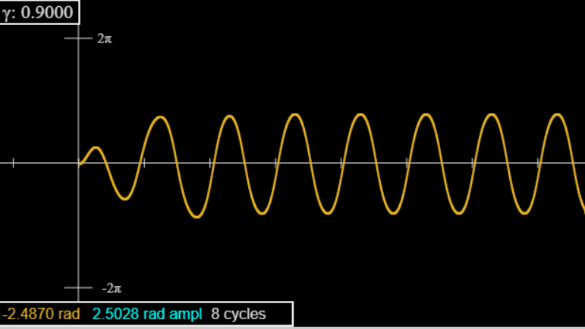For $\gamma =0.9,$ the oscillation (after brief initial transients) looks like a sine wave, although it’s slightly flatter, and notice the amplitude (turquoise in box) is greater than $\pi /2,$ the positive and negative swings are equal in magnitude to five figure accuracy after five or so oscillations.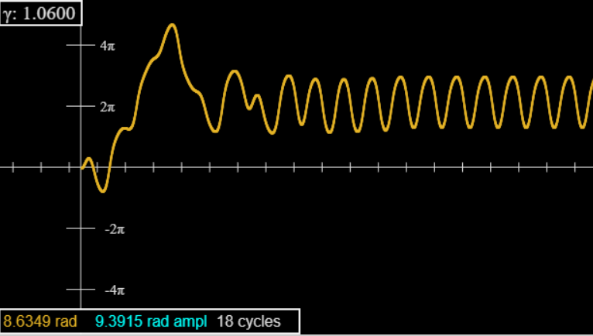For $\gamma =1.06,$ there is a larger initial transient, vaguely resembling the three-cycle we’ll meet shortly.  In fact, this can be suppressed by starting at $\phi =-\pi /2,$ but there are still transients: the peaks are not uniform to five-figure accuracy until about forty cycles.

For $\gamma =1.0662,$ there are very long transients: not evident on looking at the graph, but revealed by monitoring the amplitude (turquoise figures in the box on the graph) which reads the value at the latest extremum.

To get past these transients, set the applet speed to 50 (this does not affect accuracy, only prints a lot less dots), run 4500 cycles (won’t take long!) then pause and go to speed = 1.  You will find the peaks now all have the same height to the five-figure accuracy displayed.

Now repeat for $\gamma =1.0663$ (use the button to nudge up by one in that last digit).  Go at speed 50 to 3000 cycles, then you’ll find on pausing and going back to speed 1 that the peaks have period 2, with a 0.01 radian difference, approximately.  But this is a false alarm: it’s an incredibly long transient. The system finally settles at period 1 (to five figures) after 12,000 cycles.  We are apparently right at the bifurcation point.

Going to 1.0664 and beyond you’ll see both peaks and dips separating, for a well-defined period 2, which lasts until 1.0792.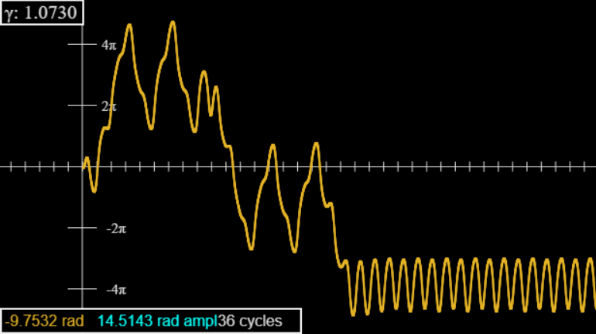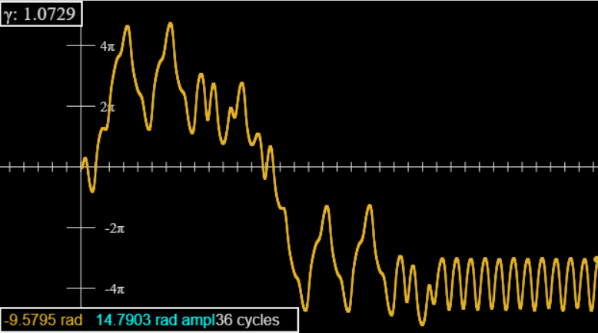For $\gamma =1.0793,$ the period doubles again: successive peaks are now of order 0.4 radians apart, but successive “high” peaks are suddenly about 0.01 radians apart.

For $\gamma =1.0821$ there is a further doubling to an 8 cycle, then at 1.0827 to a 16 cycle.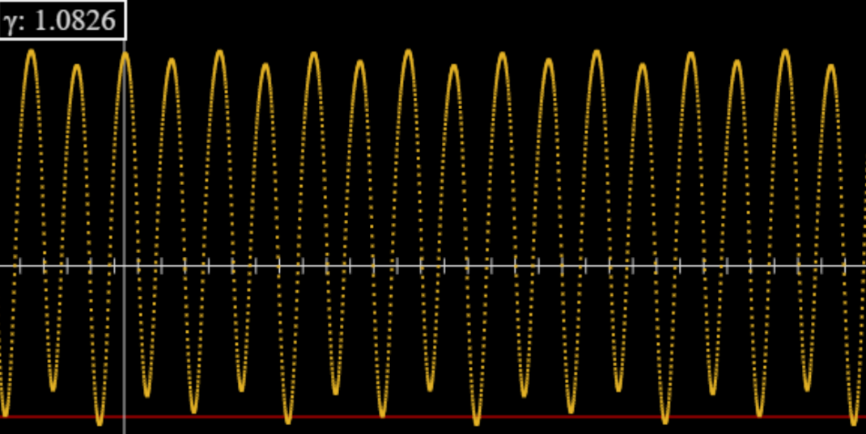Look at the graph for 1.0826: in particular, the faint red horizontal line near the bottom. Every fourth dip goes below the line, but the next lowest dips alternate between touching the line and not quite reaching it.  This is the 8 cycle.

The intervals between successive doublings decrease by the factor $\delta =4.6692,$ found universally in period doubling cascades, and called the Feigenbaum number, after its discoverer. Our five-figure accuracy is too crude to follow this sequence further, but we can establish (or at least make very plausible!) that beyond the geometric series limit at ${\gamma }_{c}=1.0829$ there is no periodicity, the system is chaotic. (Of course, the values of $\gamma$ depend on the chosen damping parameter, etc., only the ratio of doubling intervals is universal.)  However, the full picture is incredibly complex: there are further intervals of periodicity, for example a 6 cycle at $\gamma =1.0845,$ pictured below.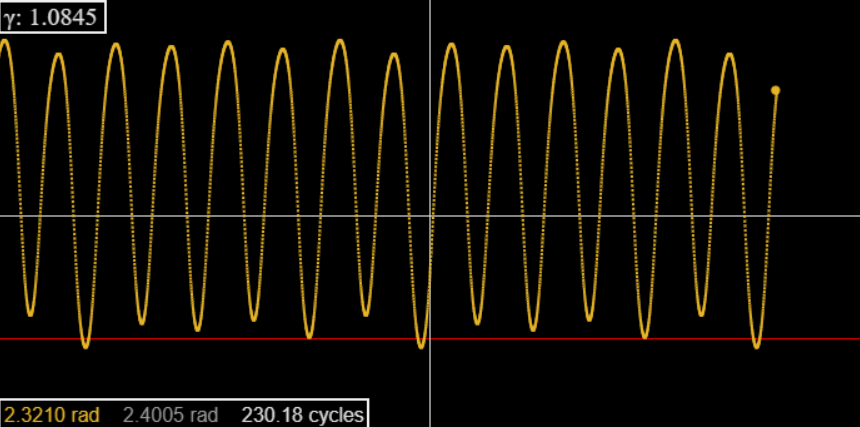### Different Attractors

The periodic solutions described above are called “attractors”: configurations where the system settles down after initially wandering around.

Clearly the attractors change with the driving strength, what is less obvious is that they may be different for different initial conditions.  Taylor shows that for $\gamma =1.077$ taking $\phi \left(0\right)=0$ gives a 3-cycle after transients, but $\phi \left(0\right)=-\pi /2$ gives a 2-cycle. (Easily checked with the applet!)

Looking at the initial wanderings, which can be quite different for very small changes in the driving strength (compare 1.0730 to 1.0729 and 1.0731, use speed 5, it doesn’t affect the accuracy). But you can see these initial wanderings include elements from both attractors.

Exercise:  Use the applet to find at what angle the transition from one attractor to the other takes place.  And, explore what happens over a range of driving strengths.

These are the simplest attractors: there are far more complex entities, called strange attractors, we may discuss later.

Exercises:  Try different values of the damping constant and see how this affects the bifurcation sequence.